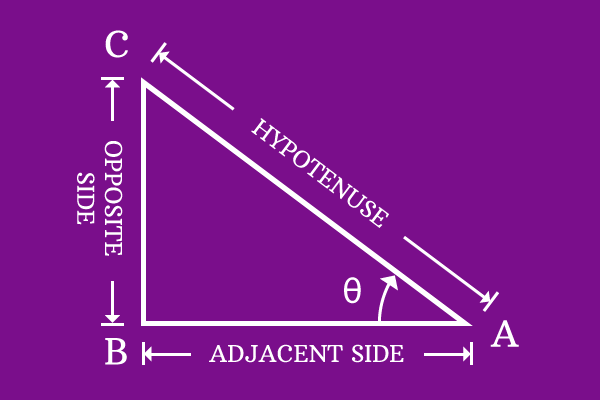# Trigonometric Ratio

## Definition

The ratio of lengths of any two of three sides of a right angled triangle at an angle is called trigonometric ratio.A right angled triangle contains three sides and a ratio is calculated by taking lengths of any two sides from three sides.

For example:

$$\frac{Length \, of \, Opposite \, side}{Length \, of \, Hypotenuse}$$

The possible ratios of three sides in a triangle by taking two sides each, are $3!$ which means $6$ ratios are possible.

### List of Ratios

Each trigonometric ratio is represented by a name to avoid complexity in expressing the ratios in terms of lengths of any two sides. Here is the list of $6$ trigonometric ratios and their definitions with represented names.

1

#### SineThe ratio of length of opposite side to length of hypotenuse at an angle of right angled triangle is called sine.
2

#### CosineThe ratio of length of adjacent side to length of hypotenuse at an angle of right angled triangle is called cosine.
3

#### TangentThe ratio of length of opposite side to length of adjacent side at an angle of right angled triangle is called tangent.
4

#### CotangentThe ratio of length of adjacent side to length of opposite side at an angle of right angled triangle is called cotangent.
5

#### SecantThe ratio of length of hypotenuse to length of adjacent side at an angle of right angled triangle is called secant.
6

#### CosecantThe ratio of length of hypotenuse to length of opposite side at an angle of right angled triangle is called cosecant.
Latest Math Topics
Latest Math Problems
Email subscription
Math Doubts is a best place to learn mathematics and from basics to advanced scientific level for students, teachers and researchers. Know more